Open In App
Related Articles
• Write an Interview Experience
• Python Programs

# Python Program for Array Rotation

Here we are going to see how we can rotate array with Python code.

Array Rotation: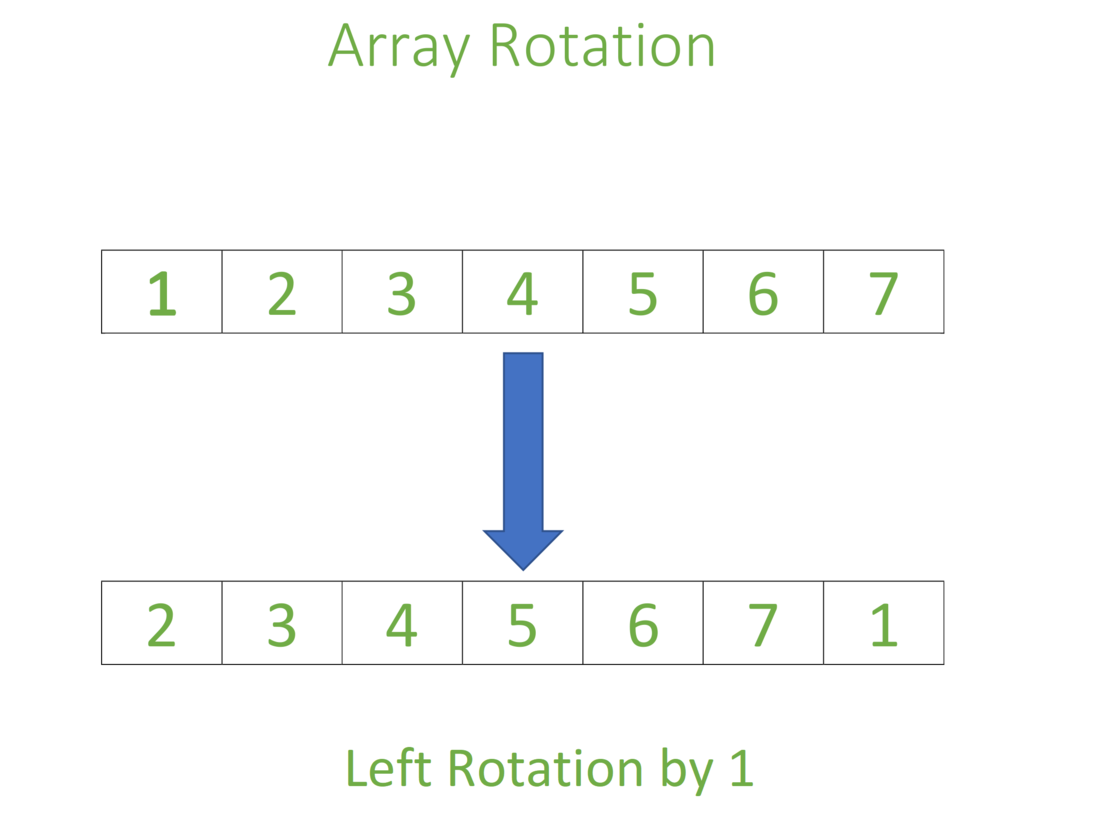## Python Program for Array Rotation Example

### Partitioning the sub arrays and reversing them

Approach:

Input arr[] = [1, 2, 3, 4, 5, 6, 7, 8], d = 1, size = 8

1) Reverse the entire list by swapping first and last numbers

i.e start=0, end=size-1

2) Partition the first subarray and reverse the first subarray, by swapping first and last numbers.

i.e start=0, end=size-d-1

3) Partition the second subarray and reverse the second subarray, by swapping first and last numbers.

i.e start=size-d, end=size-1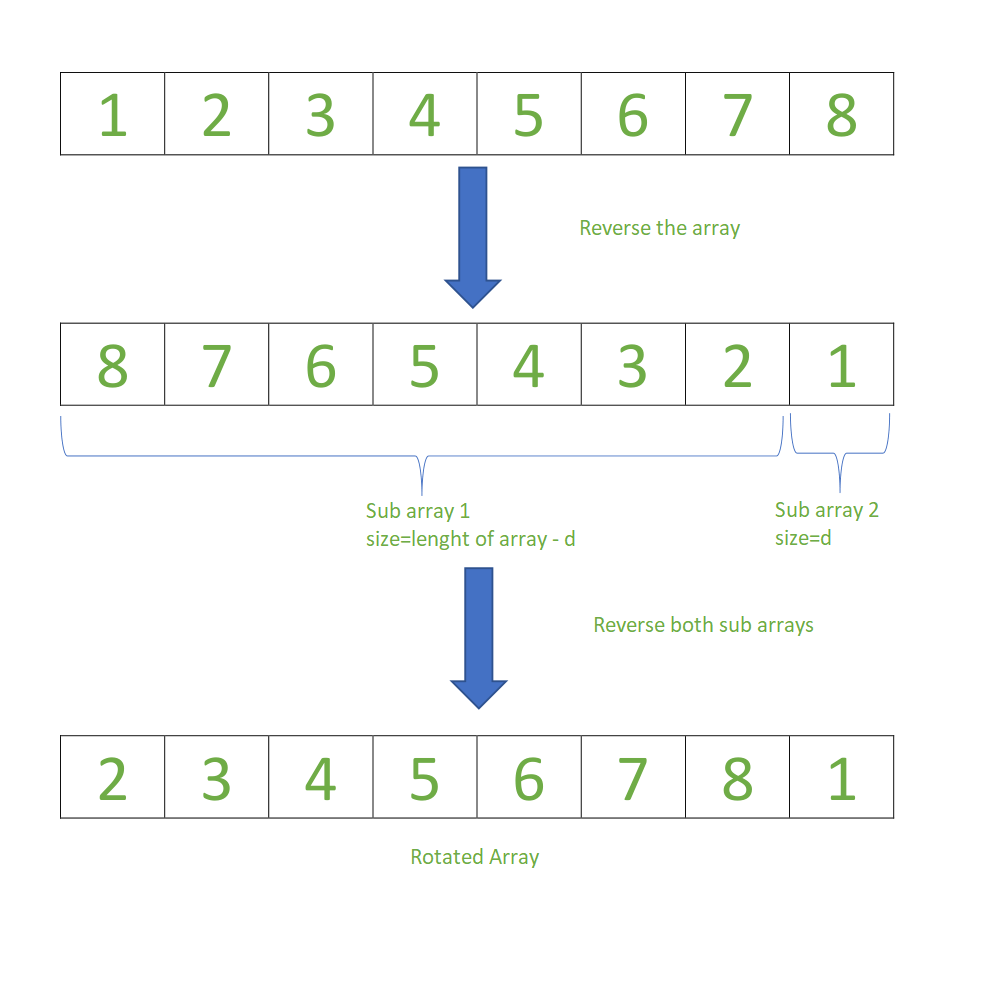Example:

## Python3

 `# Python program to left-rotate the given array` `# Function reverse the given array``# by swapping first and last numbers.`  `def` `reverse(start, end, arr):` `    ``# No of iterations needed for reversing the list``    ``no_of_reverse ``=` `end``-``start``+``1` `    ``# By incrementing count value swapping``    ``# of first and last elements is done.``    ``count ``=` `0``    ``while``((no_of_reverse)``/``/``2` `!``=` `count):``        ``arr[start``+``count], arr[end``-``count] ``=` `arr[end``-``count], arr[start``+``count]``        ``count ``+``=` `1``    ``return` `arr` `# Function takes array, length of``# array and no of rotations as input`  `def` `left_rotate_array(arr, size, d):` `    ``# Reverse the Entire List``    ``start ``=` `0``    ``end ``=` `size``-``1``    ``arr ``=` `reverse(start, end, arr)` `    ``# Divide array into twosub-array``    ``# based on no of rotations.``    ``# Divide First sub-array``    ``# Reverse the First sub-array``    ``start ``=` `0``    ``end ``=` `size``-``d``-``1``    ``arr ``=` `reverse(start, end, arr)` `    ``# Divide Second sub-array``    ``# Reverse the Second sub-array``    ``start ``=` `size``-``d``    ``end ``=` `size``-``1``    ``arr ``=` `reverse(start, end, arr)``    ``return` `arr`  `arr ``=` `[``1``, ``2``, ``3``, ``4``, ``5``, ``6``, ``7``, ``8``]``size ``=` `8``d ``=` `1``print``(``'Original array:'``, arr)` `# Finding all the symmetric rotation number``if``(d <``=` `size):``    ``print``(``'Rotated array: '``, left_rotate_array(arr, size, d))``else``:``    ``d ``=` `d ``%` `size``    ``print``(``'Rotated array: '``, left_rotate_array(arr, size, d))` `# This code contributed by SR.Dhanush`

Output

```Original array: [1, 2, 3, 4, 5, 6, 7, 8]
Rotated array:  [2, 3, 4, 5, 6, 7, 8, 1]```

Time Complexity: O(log10(Half no of elements presents in the given array)).

Auxiliary Space: O(1).

## Python Program for Array Rotation Using temp array

Write a function rotate(ar[], d, n) that rotates arr[] of size n by d elements.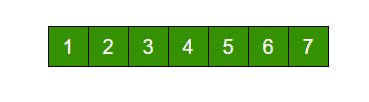Rotation of the above array by 2 will make array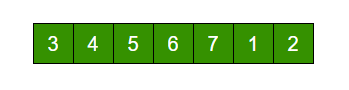```Input arr[] = [1, 2, 3, 4, 5, 6, 7], d = 2, n =7
1) Store d elements in a temp array
temp[] = [1, 2]
2) Shift rest of the arr[]
arr[] = [3, 4, 5, 6, 7, 6, 7]
3) Store back the d elements
arr[] = [3, 4, 5, 6, 7, 1, 2]```

## Python3

 `# function to rotate array by d elements using temp array``def` `rotateArray(arr, n, d):``    ``temp ``=` `[]``    ``i ``=` `0``    ``while` `(i < d):``        ``temp.append(arr[i])``        ``i ``=` `i ``+` `1``    ``i ``=` `0``    ``while` `(d < n):``        ``arr[i] ``=` `arr[d]``        ``i ``=` `i ``+` `1``        ``d ``=` `d ``+` `1``    ``arr[:] ``=` `arr[: i] ``+` `temp``    ``return` `arr`  `# Driver function to test above function``arr ``=` `[``1``, ``2``, ``3``, ``4``, ``5``, ``6``, ``7``]``print``(``"Array after left rotation is: "``, end``=``' '``)``print``(rotateArray(arr, ``len``(arr), ``2``))`

Output

`Array after left rotation is:  [3, 4, 5, 6, 7, 1, 2]`

Time complexity: O(n)
Auxiliary Space: O(d)

## Python Program for Array Rotation Using Rotate one by one

```leftRotate(arr[], d, n)
start
For i = 0 to i < d
Left rotate all elements of arr[] by one
end```

To rotate by one, store arr in a temporary variable temp, move arr to arr, arr to arr …and finally temp to arr[n-1]

Let us take the same example arr[] = [1, 2, 3, 4, 5, 6, 7], d = 2

Rotate arr[] by one 2 times We get [2, 3, 4, 5, 6, 7, 1] after first rotation and [ 3, 4, 5, 6, 7, 1, 2] after second rotation.

## Python3

 `#Function to left rotate arr[] of size n by d*/``def` `leftRotate(arr, d, n):``    ``for` `i ``in` `range``(d):``        ``leftRotatebyOne(arr, n)` `#Function to left Rotate arr[] of size n by 1*/``def` `leftRotatebyOne(arr, n):``    ``temp ``=` `arr[``0``]``    ``for` `i ``in` `range``(n``-``1``):``        ``arr[i] ``=` `arr[i``+``1``]``    ``arr[n``-``1``] ``=` `temp``        `  `# utility function to print an array */``def` `printArray(arr,size):``    ``for` `i ``in` `range``(size):``        ``print` `(``"%d"``%` `arr[i],end``=``" "``)` ` ` `# Driver program to test above functions */``arr ``=` `[``1``, ``2``, ``3``, ``4``, ``5``, ``6``, ``7``]``leftRotate(arr, ``2``, ``7``)``printArray(arr, ``7``)` `# This code is contributed by Shreyanshi Arun`

Output

`3 4 5 6 7 1 2 `

Time complexity : O(n * d)
Auxiliary Space : O(1)

### Python Program for Array Rotation Using 4 Juggling Algorithm

This is an extension of method 2. Instead of moving one by one, divide the array in different sets
where number of sets is equal to GCD of n and d and move the elements within sets.
If GCD is 1 as is for the above example array (n = 7 and d =2), then elements will be moved within one set only, we just start with temp = arr and keep moving arr[I+d] to arr[I] and finally store temp at the right place.
Here is an example for n =12 and d = 3. GCD is 3 and

```Let arr[] be {1, 2, 3, 4, 5, 6, 7, 8, 9, 10, 11, 12}

a)    Elements are first moved in first set – (See below diagram for this movement```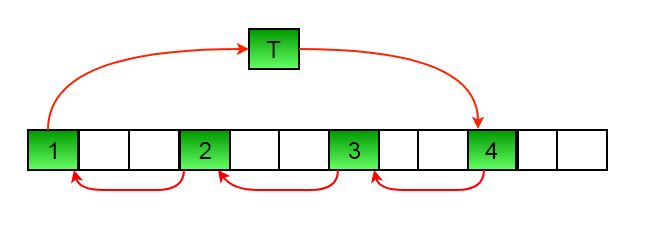```          arr[] after this step --> {4 2 3 7 5 6 10 8 9 1 11 12}

b)    Then in second set.
arr[] after this step --> {4 5 3 7 8 6 10 11 9 1 2 12}

c)    Finally in third set.
arr[] after this step --> {4 5 6 7 8 9 10 11 12 1 2 3}```

## Python3

 `# Function to left rotate arr[] of size n by d``def` `leftRotate(arr, d, n):``    ``for` `i ``in` `range``(gcd(d, n)):` `        ``# move i-th values of blocks``        ``temp ``=` `arr[i]``        ``j ``=` `i``        ``while` `1``:``            ``k ``=` `j ``+` `d``            ``if` `k >``=` `n:``                ``k ``=` `k ``-` `n``            ``if` `k ``=``=` `i:``                ``break``            ``arr[j] ``=` `arr[k]``            ``j ``=` `k``        ``arr[j] ``=` `temp` `# UTILITY FUNCTIONS``# function to print an array`  `def` `printArray(arr, size):``    ``for` `i ``in` `range``(size):``        ``print``(``"%d"` `%` `arr[i], end``=``" "``)` `# Function to get gcd of a and b`  `def` `gcd(a, b):``    ``if` `b ``=``=` `0``:``        ``return` `a``    ``else``:``        ``return` `gcd(b, a ``%` `b)`  `# Driver program to test above functions``arr ``=` `[``1``, ``2``, ``3``, ``4``, ``5``, ``6``, ``7``]``leftRotate(arr, ``2``, ``7``)``printArray(arr, ``7``)`

Output

`3 4 5 6 7 1 2 `

Time complexity : O(n)
Auxiliary Space : O(1)

Another Approach : Using List slicing

## Python3

 `# Python program using the List``# slicing approach to rotate the array``def` `rotateList(arr,d,n):``  ``arr[:]``=``arr[d:n]``+``arr[``0``:d]``  ``return` `arr``# Driver function to test above function``arr ``=` `[``1``, ``2``, ``3``, ``4``, ``5``, ``6``]``print``(arr)``print``(``"Rotated list is"``)``print``(rotateList(arr,``2``,``len``(arr))) ` `# this code is contributed by virusbuddah`

Output

```[1, 2, 3, 4, 5, 6]
Rotated list is
[3, 4, 5, 6, 1, 2]```

If array needs to be rotated by more than its length then mod should be done.

For example: rotate arr[] of size n by d where d is greater than n. In this case d%n should be calculated and rotate by the result after mod.

Time complexity : O(n) where n is size of given array
Auxiliary Space : O(1)

Please refer complete article on Program for array rotation for more details!